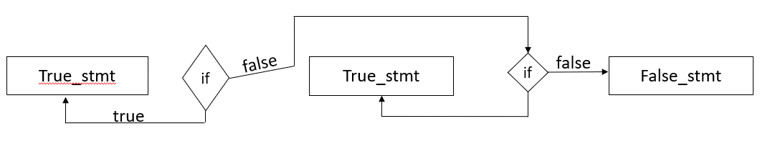In this article, we are going to discuss how to use python ternary operator and implementing nested-if-else with ternary operator along with basic examples

Ternary Operators are also called as Conditional Operators which are decision-making statements. These Ternary operators have the same functionality as if-else statements, the only difference is the lines of code. In the general approach of `if-else` statements, there may be greater than 4 lines of code, while we only use a single line in the ternary operator. However, the result will not be affected based on the approach.

## Syntax:

[True_stmt] if [cond] else [false_stmt]

## Ternary Operator Example

In the below example, the idea is to check the value of variable ‘a’ after checking the value if the value of a is 10, we print “a is 10” otherwise “a is not 10”.

First, the compiler checks the condition at the `if statement`, if the condition is true then the true statement is executed otherwise false statement is executed.
As, the condition at the if the statement is true, the true statement gets printed I.e. a is 10.

``````a=10
print("a is 10") if a==10 else print("a is not 10")
``````

Output:

``a is 10 ``

## Ternary if else

In the below example, the idea is to check whether the value of sum is less than or greater than 50. num1 and num2 are initialised with values 100 and 20 respectively which are assigned to sum variable by adding them. After performing sum operation on the given numbers, the compiler checks for if condition, if the condition is true then true statement gets executed otherwise false statement gets executed.

As sum is greater than 50, false statement gets executed.

``````num1 = 100
num2 = 20
sum = num1+num2
print("sum is less than 50") if sum<50 else print("sum is greater than 50")
``````

Output:

``sum is greater than 50 ``

In the above two examples, the idea of `if-else` statements are explained, In the below example observe the implementation of `nested-if-else` using the ternary operator in python.

## Ternary nested if else

Generally, `nested-if-else` would first check for the first if condition and if the condition is true then it executes print statement otherwise else block gets executed i.e. it checks for the next if condition and prints true statement if the condition is true otherwise it prints the false statement.In the below code, the compiler first checks the if condition, if the condition is true then it prints the true statement, if the condition is false then it checks for the next if condition in else block and prints true statement if the condition is true otherwise prints the false statement.

``````num1 = 1000
num2 = 100
print("num1 >num2") if num1>num2 else print("num2>num1") if num1``````

Output:

``num1 >num2 ``

Let us see another example using the above approach, consider the numbers 93,98,88. Our task is to find the biggest number among the given three numbers using the ternary operator.

In the below code we have given values to num1,num2,num3, for finding the biggest among these three we have used two if conditions, the first if condition checks the condition if the condition is true then it prints the true statement and then it checks for the next if condition and checks the condition and prints the appropriate statement based on the condition

``````num1 = 93
num2 = 98
num3 = 88
print("num1 is greater") if num1>num2 and num1>num3 else print("num2 is greater") if num2>num3 else print("num3 is greater")
``````

Output:

``num2 is greater``

## Benefits of using Ternary operator

• Reduces the lines of code
• Increases the readability of code

## References:

Happy Learning 🙂